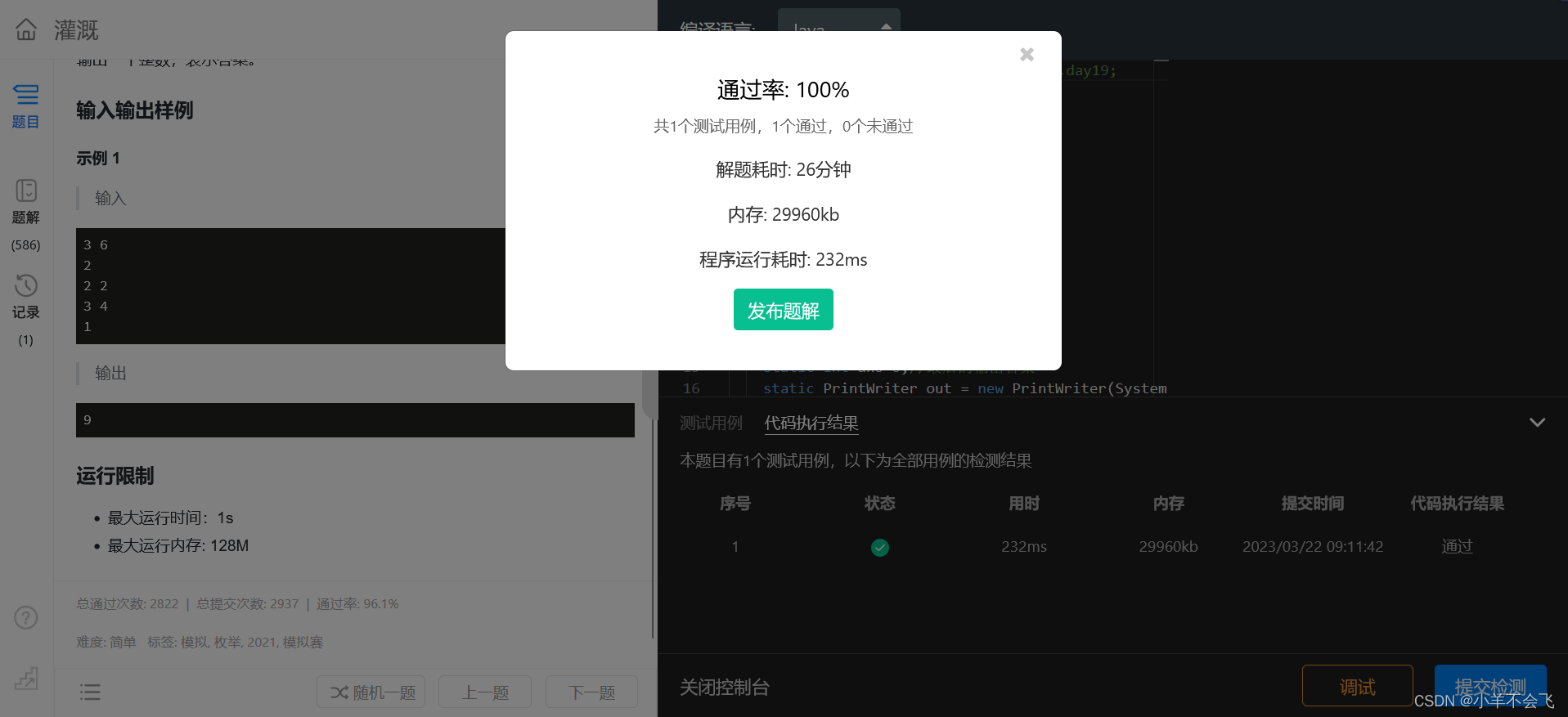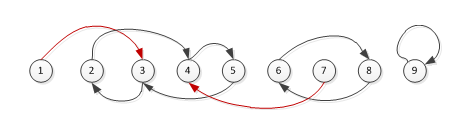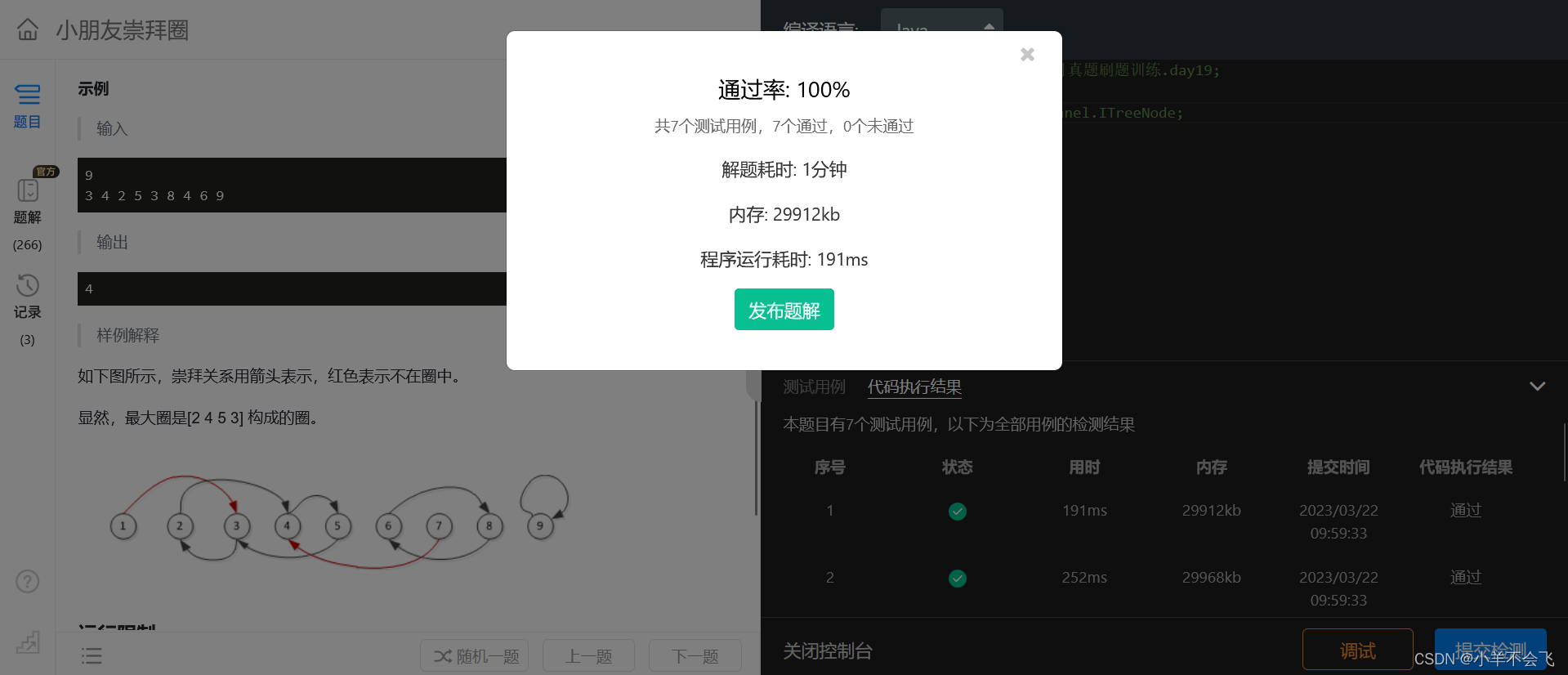# 第 1 题：灌溉_BFS板子题

### 输入输出样例

``````3 6
2
2 2
3 4
1
``````

``````9
``````

• 最大运行时间：1s
• 最大运行内存: 128M

## 代码：

``````package 第十四届蓝桥杯三月真题刷题训练.day19;

import java.io.*;
import java.util.Queue;

/**
* @author yx
* @date 2023-03-22 8:29
*/
public class 灌溉_BFS模板题 {
static int[] X = {0, 0, -1, 1};
static int[] Y = {1, -1, 0, 0};
static int[][] map;
static int ans=0;//最后的输出答案
static PrintWriter out = new PrintWriter(System.out);
static StreamTokenizer in = new StreamTokenizer(ins);

/**
* 输入
* in.nextToken()
* int a= (int)in.nval;
* <p>
* 输出
* out.print();
* out.flush();
* <p>
* 读文件：
* if (s == null)break;读取文件终止的语句
**/
public static void main(String[] args) throws IOException {
in.nextToken();
int n = (int) in.nval;
in.nextToken();
int m = (int) in.nval;
in.nextToken();
int t = (int) in.nval;
//初始就有t个出水点
ans=t;
//存储出水管的（x,y）
int[][] XY = new int[t];
for (int i = 0; i < 2; i++) {
XY[i] = Integer.parseInt(sp);
XY[i] = Integer.parseInt(sp);
}
in.nextToken();
int k = (int) in.nval;
map = new int[n][m];
//队列
//对方格进行初始化
for (int i = 0; i < t; i++) {
map[XY[i] - 1][XY[i] - 1] = 1;
//把洒水点入队
queue.offer(new int[]{XY[i] - 1, XY[i] - 1});
}
//不能超出k次循环且队列不为空
while (k > 0 && !queue.isEmpty()) {
//k分钟,一个循环消耗一分钟
k--;
int length = queue.size();
for (int i = 0; i < length; i++) {
//出队
int[] nums = queue.poll();
int x = nums;
int y = nums;
//遍历四个方向
for (int j = 0; j < 4; j++) {
int newX = x + X[j];
int newY = y + Y[j];
//m行n列
if (newX < n && newX >= 0 && newY < m && newY >= 0) {
if(map[newX][newY]==0){//表示当前位置没有洒水
ans++;
map[newX][newY]=1;//对该位置赋值
//把新洒水的位置入队
queue.offer(new int[]{newX,newY});
}
}
}
}
}
out.println(ans);
out.flush();
}
}
``````## 解析：

（1）这一题是一道经典的BFS板子题，几乎不需要对板子改变什么

（2）讲一下BFS搜索的几个要点：

1. 初始化的一个二维数组Map
2. 使用队列这一数据结构，将搜过的“老点”出队，将初始的“新点”入队
3. 创建初始数组X={0，0，-1，1}，Y={1，-1，0，0}，每个点都要遍历一遍这个数组，表示可以往上下左右四个方向进行搜索
4. 对新点要进行特判（数组越界、是否搜过....)这两个特判条件是最基本的，其它条件因题而异，比如可能会更加复杂一点（是否有障碍物......）

# 第 2 题：小朋友崇拜圈_暴搜

### 输入输出样例

``````9
3 4 2 5 3 8 4 6 9
``````

``````4
``````• 最大运行时间：1s
• 最大运行内存: 256M

## 代码：

``````package 第十四届蓝桥杯三月真题刷题训练.day19;

import java.io.*;

/**
* @author yx
* @date 2023-03-22 9:46
*/
public class 小朋友崇拜圈_爆搜 {
static int[] nums;
static int max=0;
static int N;
static PrintWriter out =new PrintWriter(System.out);
static StreamTokenizer in=new StreamTokenizer(ins);
/**
* 输入
* in.nextToken()
* int a= (int)in.nval;
*
* 输出
* out.print();
* out.flush();
*
* 读文件：
* if (s == null)break;读取文件终止的语句
**/

public static void main(String[] args) throws IOException {
in.nextToken();
N=(int) in.nval;
nums=new int[N+1];
//初始化数据
for (int i = 1; i <= N; i++) {
in.nextToken();
nums[i]=(int) in.nval;
}
for (int i = 1; i <= N; i++) {
int length=dfs(i);
if(max<length){
max=length;
}
}
out.println(max);
out.flush();
}
static int dfs(int i){
//初始往下走一个位置
int key=nums[i];
int length=1;
//往下爆搜，直到起点等于终点为止
while (key!=i){
key=nums[key];
length++;
//进入死环，返回0
if(length>N){
return 0;
}
}
return length;
}
}
``````## 解析：

（1）首先我们先对数组进行初始化，每个数组里面的存储的是对应下标学号的偶像

（比如：nums=3,表示学号为1的同学崇拜的对象是学号为3的对象）

（2）其次我们遍历每一个数组元素，对其进行爆搜，此时我们需要注意一种死环的情况，比如1-->2-->3-->2-->3......一直在2和3之间绕圈圈，并且这个时候1，2，3并不能构成一个环，并且无限死循环下去，所以针对这个我们要特判一下，就这个行代码

``````//进入死环，返回0
if(length>N){
return 0;
}``````